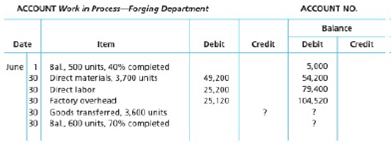Chapter 20, Problem 20.28EX

Chapter
Section
Textbook Problem

Cost per equivalent unit: average cost methodThe following information concerns production in the Forging Department for June.The forging Department uses the average cost method.a. Determine the cost per equivalent unit.b. Determine the cost of units transferred to finished Goods.c. Determine the cost of units in ending work in Process.

(a)

To determine

Process costs

It is a method of cost accounting, which is used where the production is continuous, and the product needs various processes to complete. This method is used to ascertain the cost of the product at each process or stage of production.

Equivalents units for production

The activity of a processing department in terms of fully completed units is known as equivalent units. It includes the completed units of direct materials and conversion cost of beginning work in process, units completed and transferred out, and ending work in process.

Cost per unit

Total unit cost is the cost incurred by the company to produce one unit of product. The unit cost is calculated by dividing the units produced with the total cost.

(a)

To Determine: The cost per equivalent unit.

Explanation

Calculate the number of whole units to be accounted for as shown below:

Total accounted for = Units transferred out +Ending work in process inventory=3,600units +600units=4,200units

Total accounted for is calculated by adding units transferred out and ending work in process inventory. Therefore, total accounted for 4,200 units.

Calculate the units to be assigned costs as shown below:

Assigned costs = Units transferred out +Ending work in process inventory=3,600units +600units=4,200units

Assigned costs are calculated by adding units transferred out and ending work in process inventory. Therefore, assigned costs for 4,200 units.

Calculate the number of equivalent units for production as shown below:

 Particulars Whole Units (A) Percentage to Complete (B) Units (A × B) Transferred to packing department 3,600 100% 3,600 Add: Ending work in process inventory 600 70% 420 Total 4,200 4,020

Table (1)

Equivalents units for production are calculated by adding transferred out and ending work in process inventory

(b)

To determine
The cost of units transferred to finished goods.

(c)

To determine
The cost of units in ending work in process.

Still sussing out bartleby?

Check out a sample textbook solution.

See a sample solution

The Solution to Your Study Problems

Bartleby provides explanations to thousands of textbook problems written by our experts, many with advanced degrees!

Get Started

Find more solutions based on key concepts